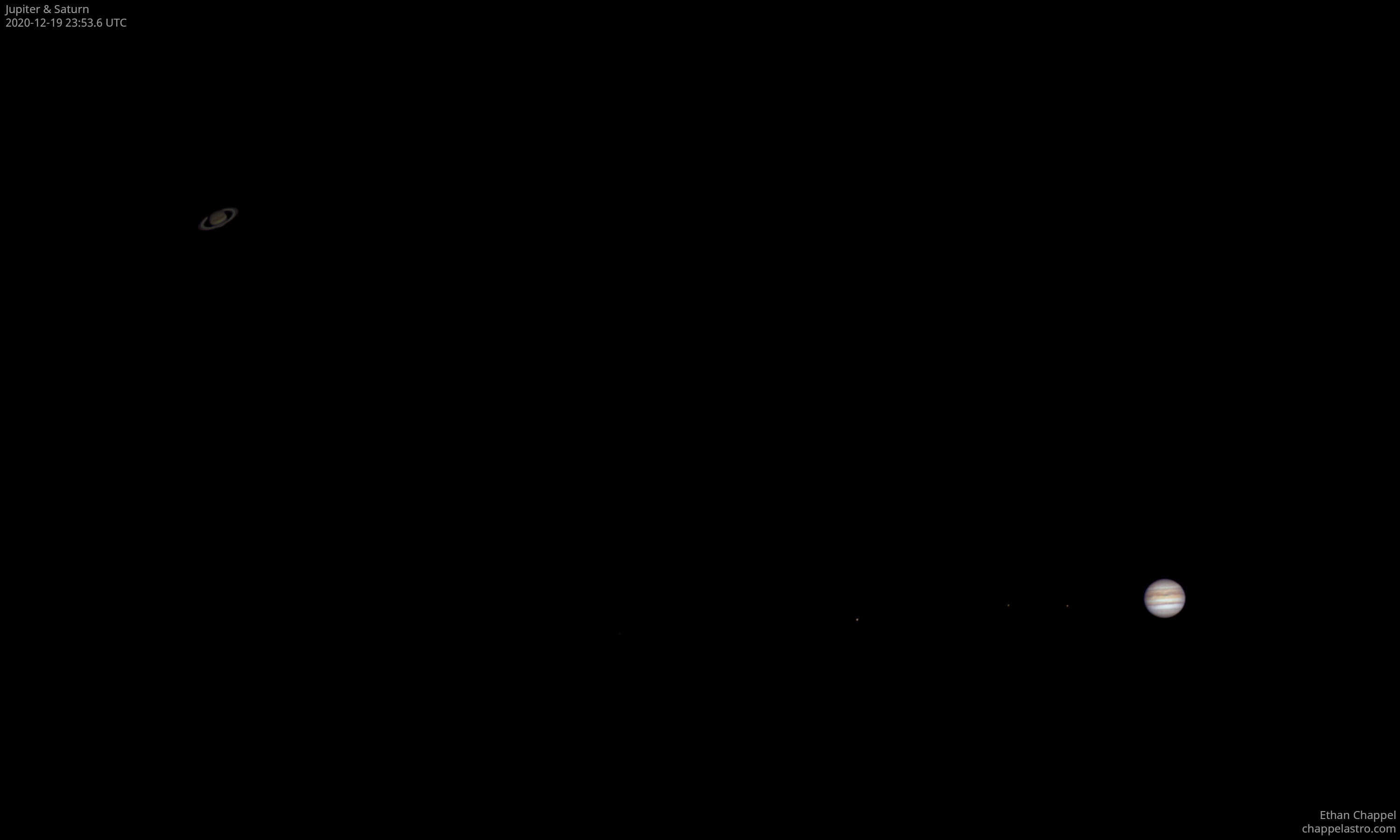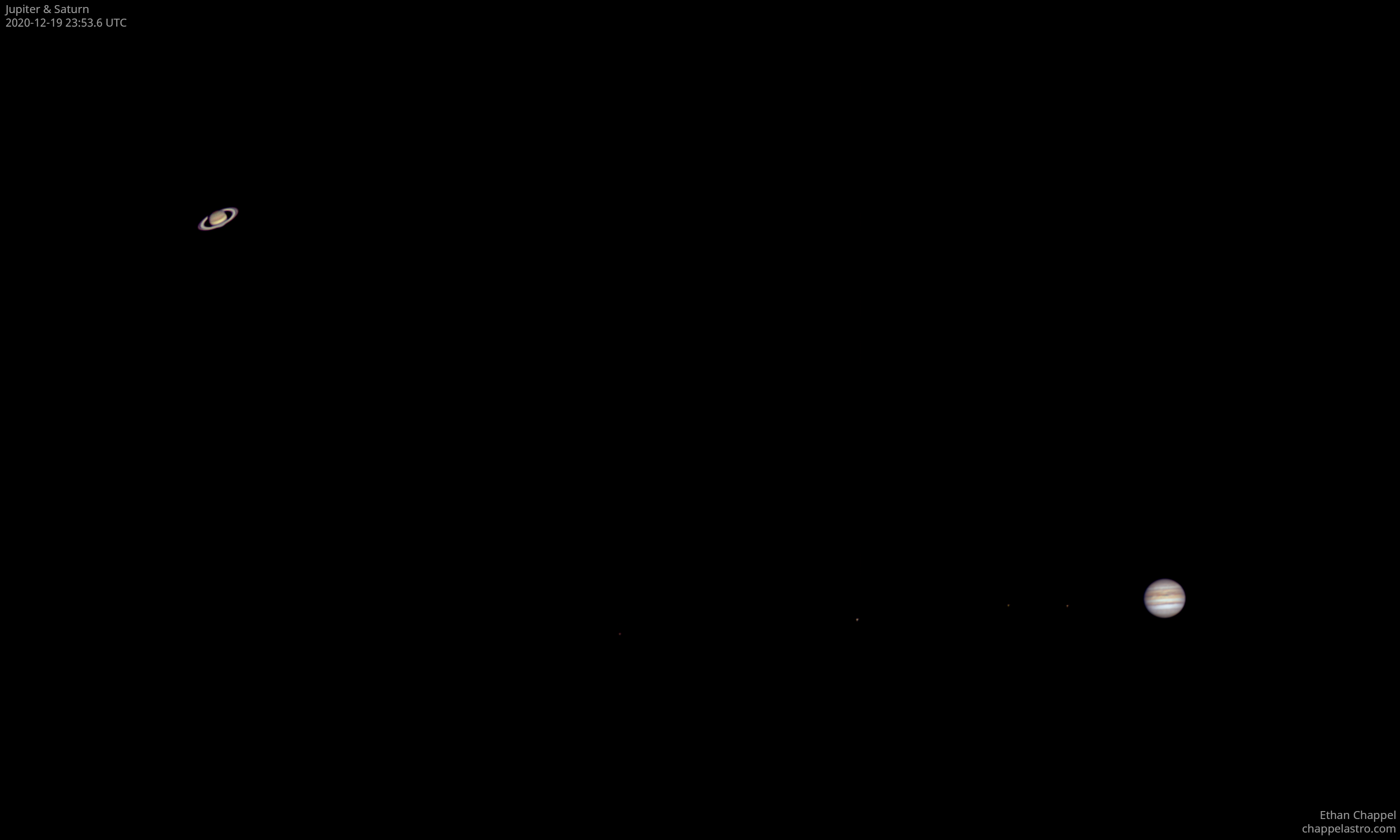# Rare Events 2020-12-19 23:53 UTC

Description

The first night I could capture Jupiter and Saturn in the same field of view using a dedicated planetary camera. All four of Jupiter's largest moons happen to be ordered by their distance from the planet. From right to left: Io, Europa, Ganymede, and Callisto.

This image shows a great example of the inverse-square law in play. While taking this photo, Jupiter was 5.1 AU from the Sun while Saturn was 10 AU. Saturn receives a quarter of the solar energy that Jupiter does, making it appear much dimmer than its inner neighbor. Our eyes can handle the difference well when looking at them through an eyepiece of a telescope, but the difference is more stark with a camera.

While the two gas giants are close together in the sky, they are physically far apart, only appearing next to each other because they and Earth are aligned in a nearly straight line. When taking this photo, Jupiter was 884 million kilometers away while Saturn was 1.8x farther at 1618 million kilometers.

Equipment

Celestron C8 EdgeHD

ZWO ASI174MM

Logs
```FireCapture v2.7 02 BETA Settings
------------------------------------
Observer=Ethan Chappel
Location=Cibolo
Scope=Celestron C8 EdgeHD
Camera=ZWO ASI174MM
Filter=B
Profile=Jupiter
Diameter=33.30"
Magnitude=-1.97
CMI=311.4° CMII=173.5° CMIII=292.3°  (during mid of capture)
FocalLength=1450mm (F/4)
Resolution=0.83"
Filename=j2020-12-19_23-56-51_b_ec.ser
Date=2020_12_19
Start=23_56_06.920
Mid=23_56_51.918
End=23_57_36.916
Start(UT)=23_56_06.920
Mid(UT)=23_56_51.918
End(UT)=23_57_36.916
Duration=89.996s
Date_format=yyyy_MM_dd
Time_format=HH_mm_ss
LT=UT
Frames captured=7472
File type=SER
Binning=1x1
ROI=1936x1216
ROI(Offset)=0x0
FPS (avg.)=83
Shutter=12.00ms
Gain=230 (57%)
AutoExposure=off
AutoGain=off
AutoHisto=75 (off)
Brightness=1
FPS=100 (off)
Gamma=50
HighSpeed=on
SoftwareGain=10 (off)
USBTraffic=80
Histogramm(min)=0
Histogramm(max)=189
Histogramm=74%
Noise(avg.deviation)=1.53
Limit=90 Seconds
Sensor temperature=86.4°F
Dark=ZWOASI174MM_1936x1216__8bit_Exp=30.00ms_T=30°C_Frames=30_2020-19-12_054553.dark
FireCapture v2.7 02 BETA Settings
------------------------------------
Observer=Ethan Chappel
Location=Cibolo
Scope=Celestron C8 EdgeHD
Camera=ZWO ASI174MM
Filter=G
Profile=Jupiter
Diameter=33.30"
Magnitude=-1.97
CMI=309.4° CMII=171.5° CMIII=290.3°  (during mid of capture)
FocalLength=1850mm (F/5)
Resolution=0.65"
Filename=j2020-12-19_23-53-36_g_ec.ser
Date=2020_12_19
Start=23_52_51.766
Mid=23_53_36.770
End=23_54_21.774
Start(UT)=23_52_51.766
Mid(UT)=23_53_36.770
End(UT)=23_54_21.774
Duration=90.008s
Date_format=yyyy_MM_dd
Time_format=HH_mm_ss
LT=UT
Frames captured=8939
File type=SER
Binning=1x1
ROI=1936x1216
ROI(Offset)=0x0
FPS (avg.)=99
Shutter=10.00ms
Gain=180 (45%)
AutoExposure=off
AutoGain=off
AutoHisto=75 (off)
Brightness=1
FPS=100 (off)
Gamma=50
HighSpeed=on
SoftwareGain=10 (off)
USBTraffic=80
Histogramm(min)=0
Histogramm(max)=185
Histogramm=72%
Noise(avg.deviation)=1.23
Limit=90 Seconds
Sensor temperature=86.4°F
Dark=ZWOASI174MM_1936x1216__8bit_Exp=30.00ms_T=30°C_Frames=30_2020-19-12_054553.dark
FireCapture v2.7 02 BETA Settings
------------------------------------
Observer=Ethan Chappel
Location=Cibolo
Scope=Celestron C8 EdgeHD
Camera=ZWO ASI174MM
Filter=R
Profile=Jupiter
Diameter=33.30"
Magnitude=-1.97
CMI=307.3° CMII=169.5° CMIII=288.3°  (during mid of capture)
FocalLength=1500mm (F/4)
Resolution=0.81"
Filename=j2020-12-19_23-50-11_r_ec.ser
Date=2020_12_19
Start=23_49_26.461
Mid=23_50_11.463
End=23_50_56.466
Start(UT)=23_49_26.461
Mid(UT)=23_50_11.463
End(UT)=23_50_56.466
Duration=90.005s
Date_format=yyyy_MM_dd
Time_format=HH_mm_ss
LT=UT
Frames captured=7472
File type=SER
Binning=1x1
ROI=1936x1216
ROI(Offset)=0x0
FPS (avg.)=83
Shutter=12.00ms
Gain=200 (50%)
AutoExposure=off
AutoGain=off
AutoHisto=75 (off)
Brightness=1
FPS=100 (off)
Gamma=50
HighSpeed=on
SoftwareGain=10 (off)
USBTraffic=80
Histogramm(min)=13
Histogramm(max)=194
Histogramm=76%
Noise(avg.deviation)=1.96
Limit=90 Seconds
Sensor temperature=86.4°F
Dark=ZWOASI174MM_1936x1216__8bit_Exp=30.00ms_T=30°C_Frames=30_2020-19-12_054553.dark
```